# 基于PyTorch深度学习 -- Part1

## 第一部分 PyTorch基础

### 一、Numpy 基础

#### 1. 生成numpy数组

##### 1.1.1 生成numpy数组–从已有数据中创建数组

1. 将列表转换成ndarray:

import numpy as np
lst1 = [3.14,2.17,0,1,2]
nd1 = np.array(lst1)
print(nd1)
print(type(nd1))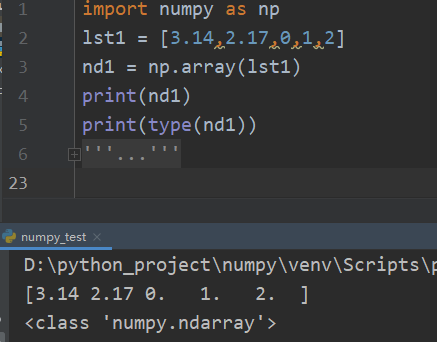1. 嵌套列表可以转换成多维ndarray:

import numpy as np
lst2 = ([3.14,2.17,0,1,2],[1,2,3,4,5])
nd2 = np.array(lst2)
print(nd2)
print(type(nd2))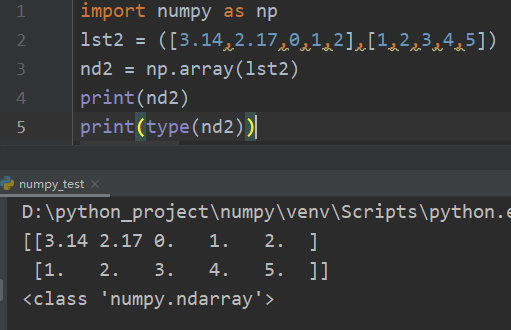##### 1.1.2 生成numpy数组–利用random模块生成数组

np.random.random 生成0到1之间的随机数
np.random.uniform 生成均匀分布的随机数
np.random.randn 生成标准正态i的随机数
np.random.randint 生成随机的整数
np.random.normal 生成正态分布
np.random.shuffle 随机打乱顺序
np.random.seed 设置随机数种子
random_sample 生成随机浮点数

import numpy as np
nd3 = np.random.random([3,3])
print(nd3)
print("nd3的形状为：",nd3.shape)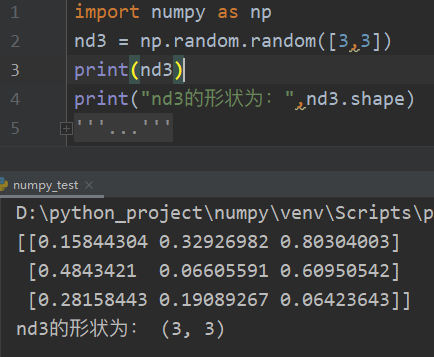import numpy as np
np.random.seed(123)    # 设置随机种子数
nd4 = np.random.randn(2,3)  # 生成标准正态的随机数
print(nd4)
np.random.shuffle(nd4)      # 随机打乱顺序
print("随机打乱后数据：")
print(nd4)
print(type(nd4))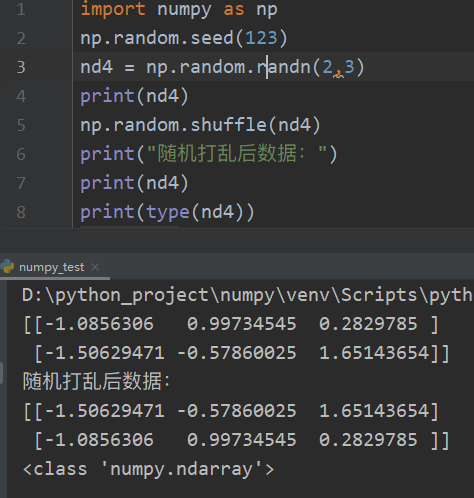##### 1.1.3 生成numpy数组 – 创建特定形状的多维数组

np.zeros、np.ones、np.diag来实现

np.zeros((3,4)) 创建3*4的元素全为0的数组
np.ones((3,4)) 创建3*4的元素全为1的数组
np.empty((2,3)) 创建2*3的空数组，空数据中的值并不为0，而是未初始化的垃圾值
np.zeros_like(ndarr) 以ndarr相同维度创建元素全为0数组
np.ones_like(ndarr) 以ndarr相同维度创建元素全为1数组
np.empty_like(ndarr) 以ndarr相同维度创建空数组
np.eye(5) 该函数用于创建一个5*5的矩阵，对角线为1，其余为0
np.full((3,5),666) 创建3*5的元素全为666的数组。666为指定值
import numpy as np
nd5 = np.zeros([3,3]) # 生成全是0的3*3矩阵
nd6 = np.ones([3,3])  # 生成全是1的3*3矩阵
nd7 = np.eye(3)       # 生成3阶的单位矩阵
nd8 = np.diag([1,2,3])# 生成3阶对角矩阵

print(nd5)
print(nd6)
print(nd7)
print(nd8)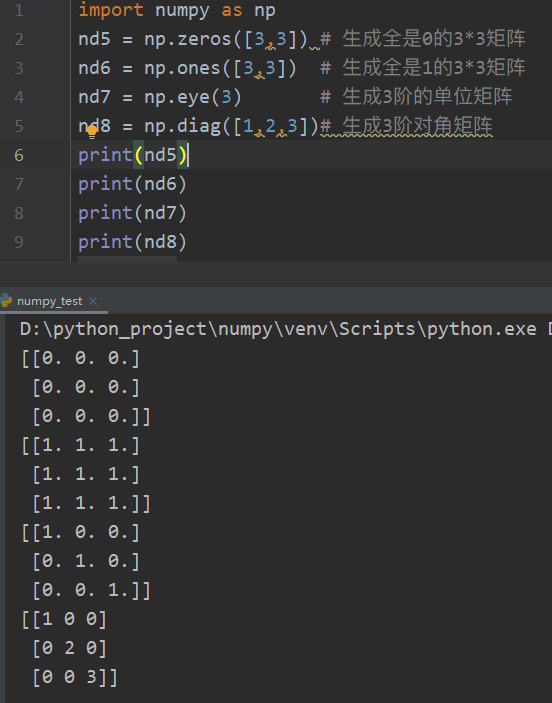import numpy as np
nd9 = np.random.random([5,5])
np.savetxt(X=nd9,fname='./test1.txt')
print(nd10)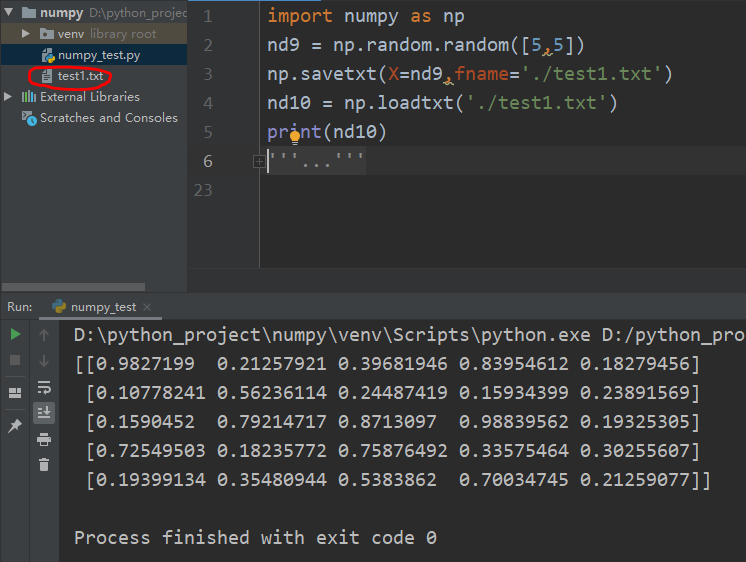##### 1.1.4 生成numpy数组 – 利用 arange、linspace函数 生成数组

arange 是 numpy模块 中的函数，格式为：

arange([start,] stop[,step,],dtype=None)


start是开始，stop是结束（start、stop用来指定范围），step用来设定步长

import numpy as np
print(np.arange(10))
print(np.arange(0,10))
print(np.arange(1,4,0.5))
print(np.arange(9,-1,-1))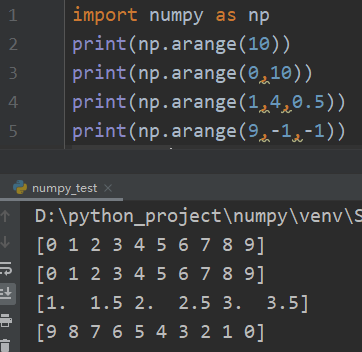linspace 是 numpy 模块 中的函数，格式为：

np.linspace(start,stop,num=50,endpoint=True,retstep=False,dtype=None)


linspace 可以根据输入的指定数据范围以及等份数量，自动生成一个线性等分向量，其中endpoint（包含终点）默认为True，等份数量num默认为50。如果将 retstep 设置为 True, 则会返回一个带步长的 ndarray。

import numpy as np
print(np.linspace(0,1,10))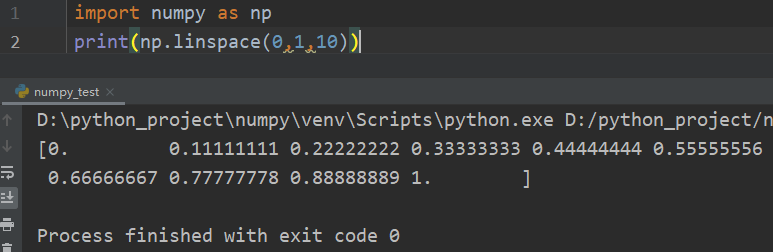### 二、Pytorch 基础

1. 定义叶子节点及算子节点：

   import torch

x = torch.tensor()

# 实现前向转播
y = torch.mul(w,x)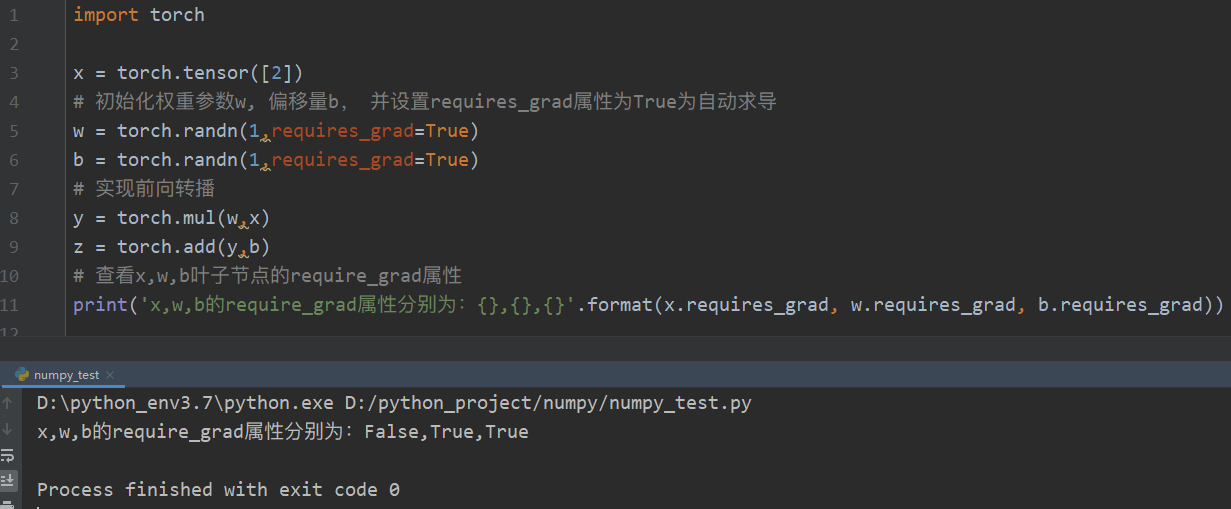2. 查看叶子节点、非叶子节点的其他属性

# 查看非叶子节点的require_grad属性

# 查看各节点是否为叶子节点
print('x,w,b,y,z的是否为叶子节点：{},{},{},{},{}'.format(x.is_leaf, w.is_leaf, b.is_leaf, y.is_leaf, z.is_leaf))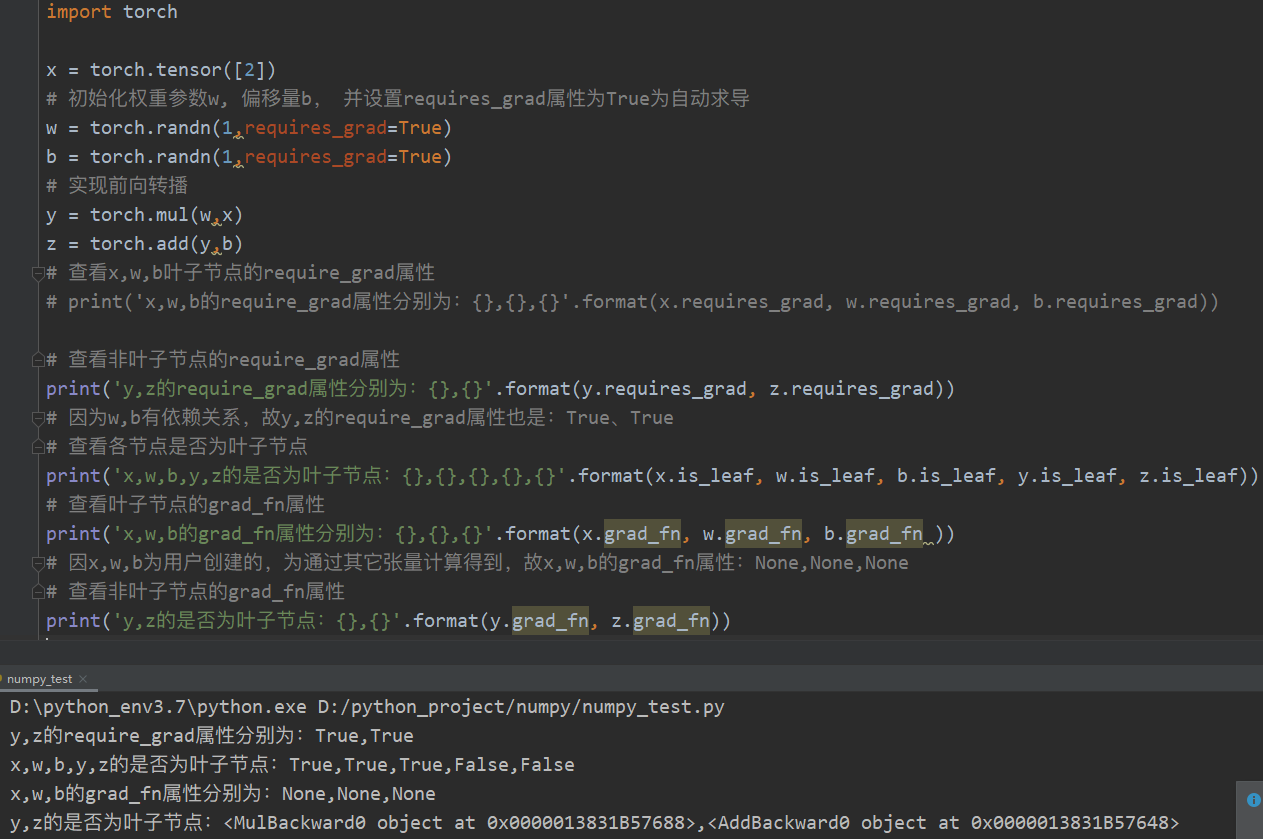3. 自动求导，实现梯度方向转播，即梯度的反向传播。

z.backward()

# 如果需要多次使用 backward, 需要修改参数 retain_graph 为 True, 此时梯度是累加的
# z.backward(retain_graph=True)

# 查看叶子节点的梯度，x是叶子节点，但它无需求导，故其梯度为None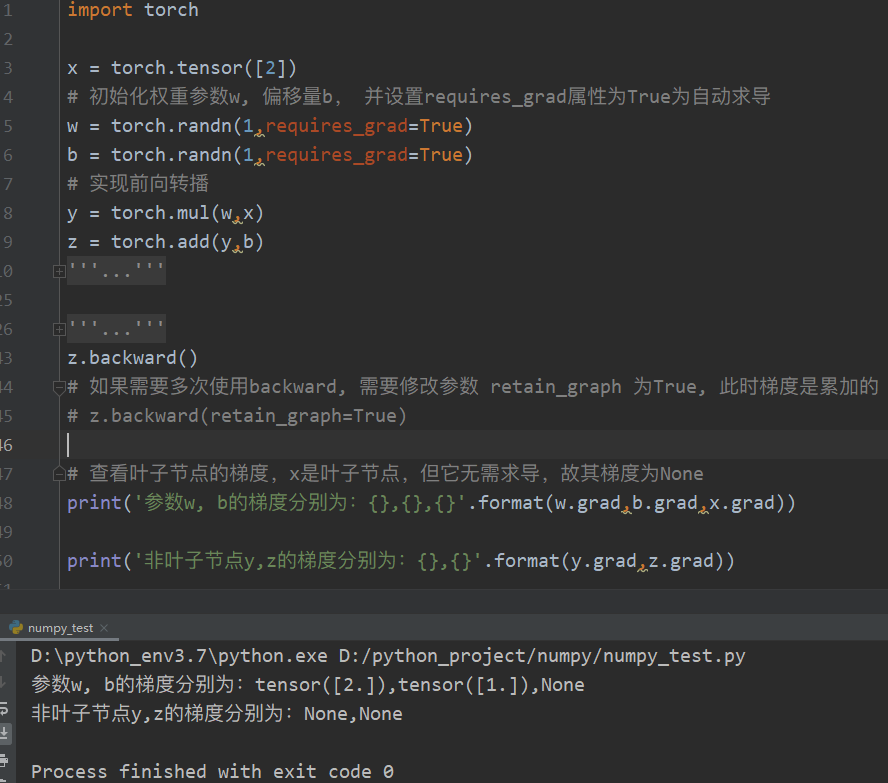backward()函数格式：

backward(gradient=None, retain_graph=None, create_graph=False)

1. 定义叶子节点及计算节点

import torch

# 定义叶子节点张量x, 形状为1*2

# 初始化雅可比矩阵
J = torch.zeros(2,2)

# 初始化目标张量，形状为1*2
y = torch.zeros(1,2)

# 定义y与x之间的映射关系
# y1=x1**2+3*x2, y2=x2**2+2*x1
y[0,0]=x[0,0]**2+3*x[0,1]
y[0,1]=x[0,1]**2+2*x[0,0]

2. 调用 backward 来获取 y 对 x 的梯度

import torch

# 定义叶子节点张量x, 形状为1*2

# 初始化雅可比矩阵
J = torch.zeros(2,2)

# 初始化目标张量，形状为1*2
y = torch.zeros(1,2)

# 定义 y 与 x 之间的映射关系
# y1=x1**2+3*x2, y2=x2**2+2*x1
y[0,0]=x[0,0]**2+3*x[0,1]
y[0,1]=x[0,1]**2+2*x[0,0]

-------下面是导致求雅可比矩阵错误的部分----------

y.backward(torch.Tensor([[1,1]]))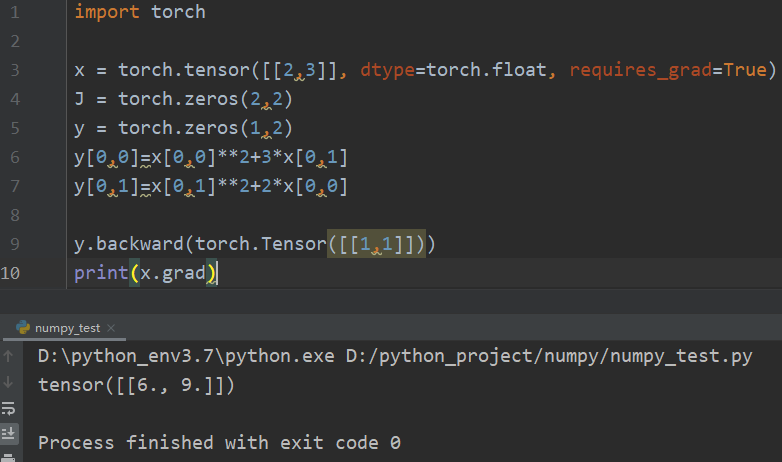import torch

# 定义叶子节点张量x, 形状为1*2

# 初始化雅可比矩阵
J = torch.zeros(2,2)

# 初始化目标张量，形状为1*2
y = torch.zeros(1,2)

# 定义 y 与 x 之间的映射关系
# y1=x1**2+3*x2, y2=x2**2+2*x1
y[0,0]=x[0,0]**2+3*x[0,1]
y[0,1]=x[0,1]**2+2*x[0,0]

--------------下述为更正部分，可与上面代码相应部分进行对比------------

y.backward(torch.Tensor([[1,0]]), retain_graph=True)

# 梯度是累加的，故需要对x的梯度清零

# 生成y2对x的梯度
y.backward(torch.Tensor([[0,1]]))

# 显示雅可比矩阵的值
print(J)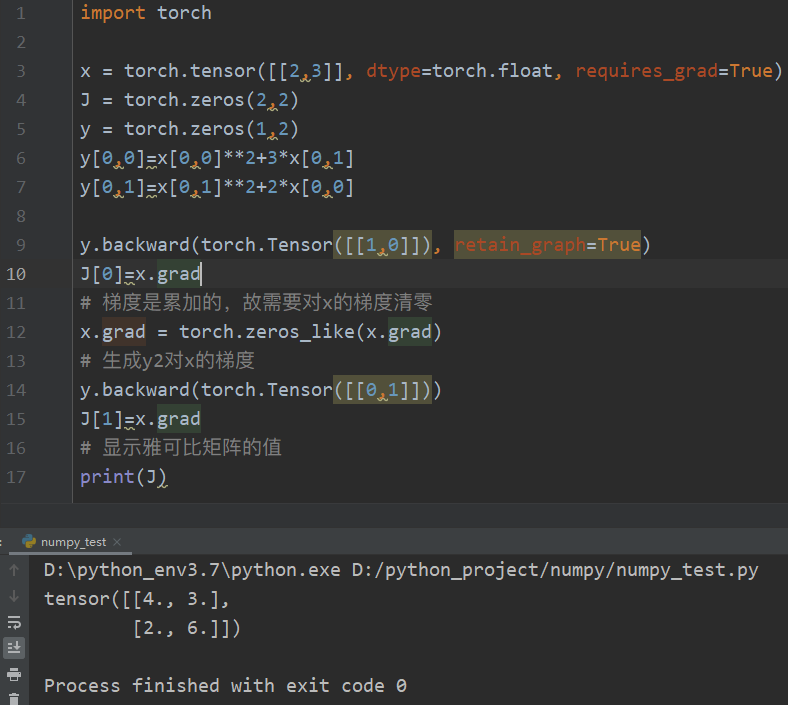#### 2.2 实现机器学习的步骤（以Numpy为例）

1. 导入需要的库

import numpy as np
from matplotlib import pyplot as plt

2. 生成 输入数据x 及 目标数据y

np.random.seed(100)
x = np.linspace(-1,1,100).reshape(100,1)
y = 3*np.power(x,2)+2+0.2*np.random.rand(x.size).reshape(100,1)

3. 查看x、y数据分布情况

plt.scatter(x,y)
plt.show()

4. 初始化权重参数

w1 = np.random.rand(1,1)
b1 = np.random.rand(1,1)

5. 训练模型

lr = 0.001
for i in range(800):
y_pred = np.power(x,2)*w1+b1

loss = 0.5*(y_pred - y)**2
loss = loss.sum()


6. 可视化结果

plt.plot(x,y_pred,'r-',label='predict')
plt.scatter(x,y,color='blue',marker='o',label='true') # true data
plt.xlim(-1,1)
plt.ylim(2,6)
plt.legend()
plt.show()
print(w1,b1)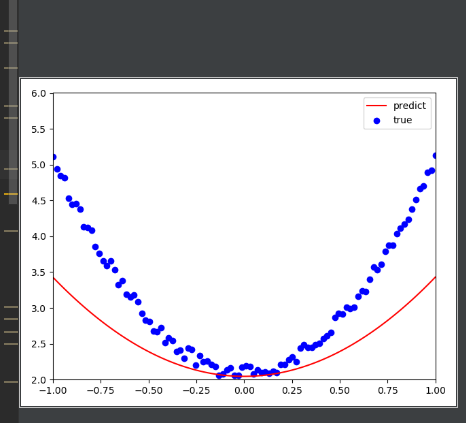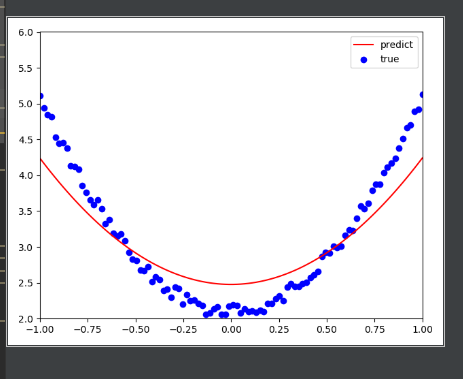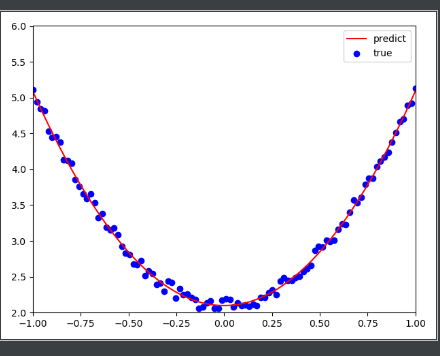### 三、PyTorch 神经网络工具箱

#### 3.1 神经网络核心组件

1. ：神经网络的基本结构，将 输入张量 转换为 输出张量（使用 Sequential( )函数的功能将网络的层组合到一起）
2. 模型：层构成的网络
3. 损失函数：参数学习的目标函数，通过最小化损失函数来学习各种参数。
4. 优化器：如何使损失函数最小，这就涉及优化器

#### 3.2 实现神经网络实例

##### – 准备数据
1. 导入必要的模块：

import numpy as np
import torch

# 导入 PyTorch 内置的 mnist数据
from torchvision.datasets import mnist

# 导入预处理模块
import torchvision.transforms as transforms
from torch.utils.data import DataLoader   # 调用 torch.utils 建立一个数据迭代器

# 导入nn及优化器
import torch.nn.functional as F
import torch.optim as optim
from torch import nn     # 利用 nn工具箱 构建神经网络模型

2. 定义一些超参数：

train_batch_size = 64
test_batch_size = 128
learning_rate = 0.01
num_epoches = 20
lr = 0.01
momentum = 0.5

3. 下载数据并对数据进行 预处理：

# 定义预处理函数，这些预处理依次放在 Compose函数 中
transforms = transforms.Compose([transforms.ToTensor(),transforms.Normalize([0.5],[0.5])])

# 下载数据，并对数据进行预处理
test_dataset = mnist.MNIST('./data',train=True, transform=transforms)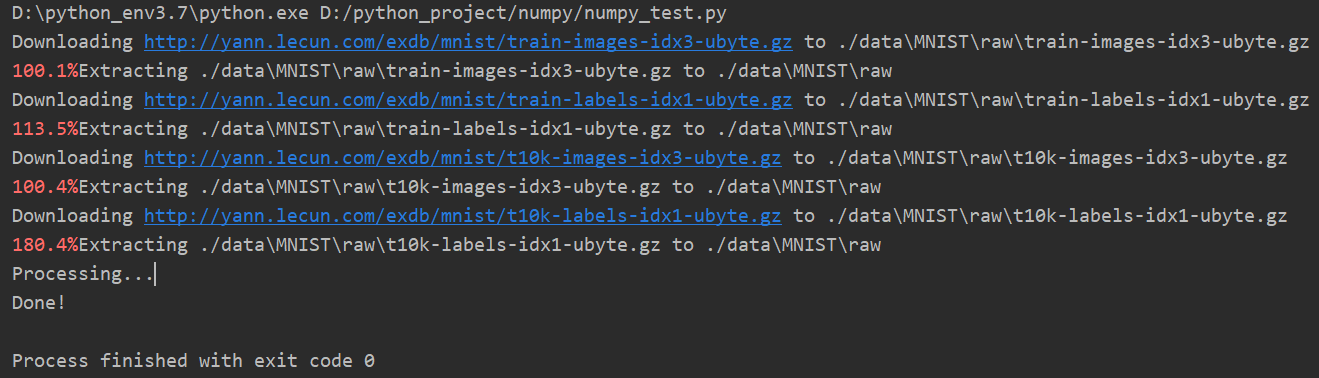##### – 可视化源数据
# 程序接上，上面的3.2.1准备数据
batch_idx,(example_data, examples_targets) = next(examples)

fig = plt.figure()
for i in range(6):
plt.subplot(2,3,i+1)
plt.tight_layout()
plt.imshow(example_data[i], cmap='gray', interpolation='none')
plt.title('Ground Truth:{}'.format(examples_targets[i]))
plt.xticks([])
plt.yticks([])
plt.show()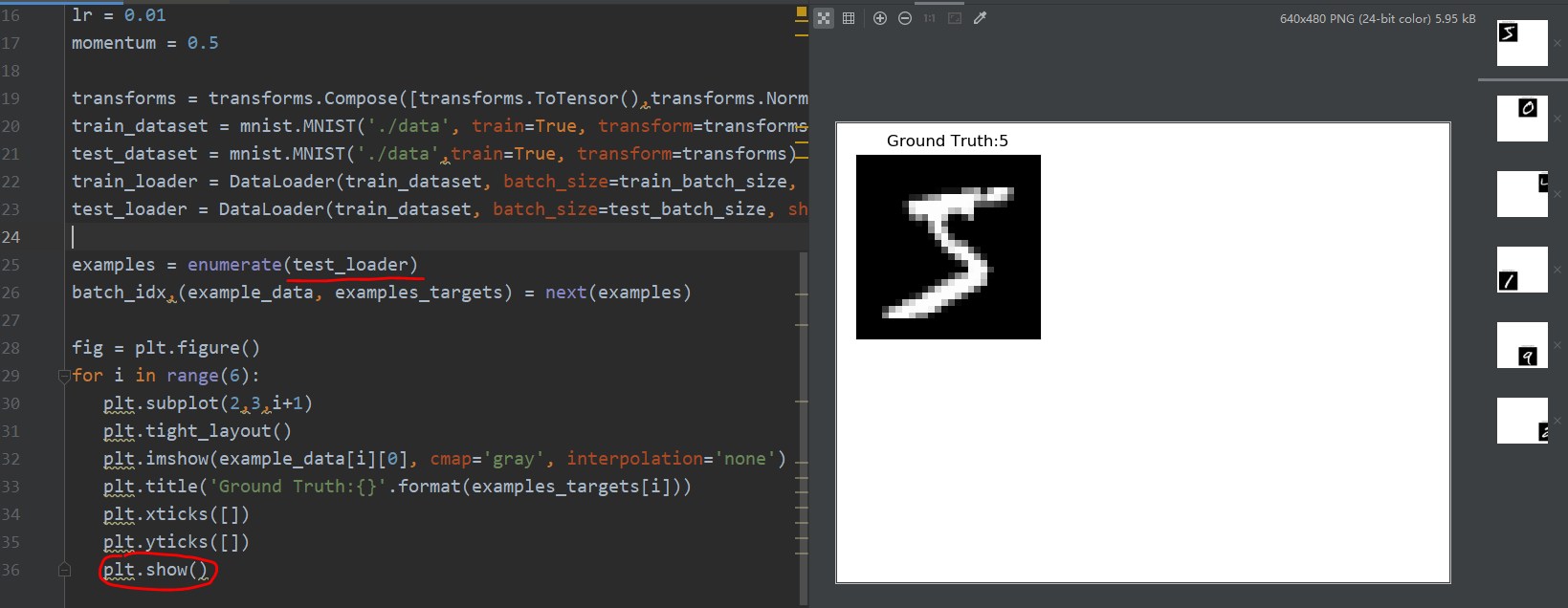##### – 构建模型
1. 构建网络

class Net(nn.Module):
'''
使用sequential构建网络，Sequential()函数的功能是将网络的层组合到一起
'''
def __init__(self, in_dim, n_hidden_1, n_hidden_2, out_dim):
super(Net,self).__init__()
self.layer1 = nn.Sequential(nn.Linear(in_dim, n_hidden_1), nn.BatchNormld(n_hidden_1))
self.layer2 = nn.Sequential(nn.Linear(n_hidden_1, n_hidden_2), nn.BatchNormld(n_hidden_1))
self.layer3 = nn.Sequential(nn.Linear(n_hidden_2, out_dim))

def forward(self, x):
x = F.relu(self.layer1(x))
x = F.relu(self.layer2(x))
x = self.layer3(x)
return  x

2. 实例化网络

   # 检测是否有可用的GPU，有则使用，否则使用CPU
device = torch.device('cuda:0' if torch.cuda.is_available() else 'cpu')

# 实例化网络
model = Net(28*28,300,100,10)
model.to(device)

# 定义损失函数和优化器
criterion = nn.CrossEntropyLoss()
optimizer = optim.SGD(model.parameters(), lr=lr, momentum = momentum)

##### – 训练模型

1. 训练模型
训练模型这里使用 for循环 进行迭代，其中包括对训练数据的 训练模型，然后用测试数据的 验证模型。

# 开始训练
losses = []
acces = []
eval_losses = []
eval_acces = []

for epoch in range(num_epoches):
train_loss = 0
train_acc = 0
model.train()

# 动态修改参数学习率
if epoch % 5 == 0:
optimizer.param_groups['lr'] *= 0.1

img = img.to(device)
label = label.to(device)
img = img.view(img.size(0), -1)

# 前向传播
out = model(img)
loss = criterion(out, label)

# 反向传播
loss.backward()
optimizer.step()
# 记录误差
train_loss += loss.item()

# 计算分类的准确率
_, pred = out.max(1)
num_correct = (pred == label).sum().item()
acc = num_correct / img.shape
train_acc += acc

# 在测试集上检验效果
eval_loss = 0
eval_acc = 0

# 将模型改为预测模式
model.eval()
img = img.to(device)
label = label.to(device)
img = img.view(img.size(0), -1)
out = model(img)
loss = criterion(out, label)

# 记录误差
eval_loss += loss.item()

# 记录准确率
_, pred = out.max(1)
num_correct = (pred == label).sum().item()
acc = num_correct / img.shape
eval_acc += acc

print('epoch:{},Train Loss: {:.4f},Train Acc: {:.4f},Test Loss: {:.4f},Test Acc: {:.4f}'.format(epoch,train_loss / len(train_loader),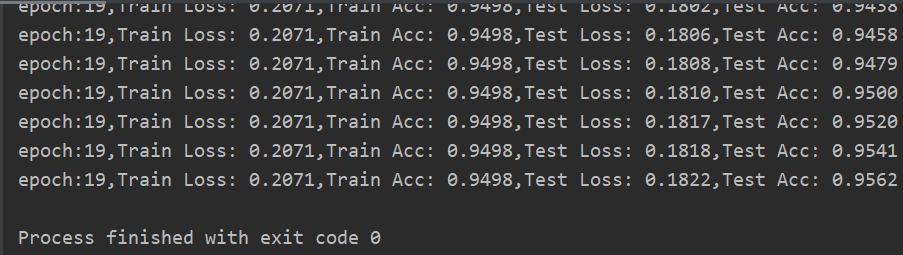# 可视化
plt.title('trainloss')
plt.plot(np.arange(len(losses)), losses)
plt.legend(['Train Loss'], loc='upper right')


#### 3.3 如何构建神经网络？

##### – 构建网络层

class Net(torch.nn.Module):
def __init__(self):
super(Net4,self).__init__()
self.conv = torch.nn.Sequential(
OrderedDict(
[
('conv1', torch.nn.Conv2d(3,32,3,1,1)),
('relu1',torch.nn.ReLU()),
('pool',torch.nn.MaxPool2d(2))
]
)
)
self.dense = torch.nn.Sequential(
OrderedDict(
[
('dense1', torch.nn.Linear(32*3*3,128)),
('relu2', torch.nn.ReLU()),
('dense2', torch.nn.Linear(128,10))
]
)
)

##### – 前向传播

forward函数的任务， 需要把 输入层、网络层、输出层链接起来，实现信息的前向传导。该函数的参数一般为输入数据，返回值为输出数据

forward函数中，有些层来自 nn.Module，也可以使用 nn.functional 定义。来自 nn.Module 的需要实例化，而使用 nn.functional 定义的可以直接使用。

#### 3.4 神经网络工具箱 nn

##### – nn.Module

nn.Module 是 nn 的一个核心数据结构，它可以是神经网络的某个层（Layer），也可以是包含多层神经网络。在实际使用中，最常见的做法是继承 nn.Module，生成自己的网络 / 层，

nn中已实现了绝大多数层，包括全连接层、损失层、激活层、卷积层、循环层 等，这些层都是 nn.Module 的子类，能够自动检测到自己的 Parameter，并将其作为学习参数，且针对GPU运行进行了 cuDNN 优化。

#### 3.5 优化器

Pytorch 常用的优化方法都封装在 torch.optim 里面，设计很灵活，可拓展为自定义的优化方法。所有的优化方法都继承了 基类optim.Optimizer，并实现了自己的优化步骤。

1. 建立优化器实例

import torch.optim as optim
optimizer = optim.SGD(model.parameters(), lr = lr, momentum=momentum)

2. 向前传播
把 输入数据 传入 神经网络Net 实例化对象model 中，自动执行 forward函数，得到out输出值，然后用out与标记label计算损失值loss

out = model(img)
loss = criterion(out,label)

3. 清空梯度
缺省情况梯度是累加的，在梯度反向传播前，先需把梯度清零。

optimizer.zero_grad()

4. 反向传播
基于损失值，把梯度进行反向传播。

loss.backward()

5. 更新参数

optimizer.step()


#### 3.6 动态修改学习率参数

1. 通过修改参数 optimizer.params_groups
2. 新建 optimizer

for epoch in range(num_epoches):

# 动态修改参数学习率
if epoch%5==0:
optimizer.param_groups['lr']*=0.1
print(optimizer.param_groups['lr'])


#### 3.7 优化器比较

PyTorch 中的优化器很多，各优化器一般有其适应的场景。

1. 导入需要的模块

import torch
import torch.utils.data as Data
import torch.nn.functional as F
import matplotlib.pyplot as plt
%matplotlib inline

% 超参数
LR = 0.01
BATCH_SIZE = 32
EPOCH = 12

2. 生成数据

# 生成训练数据
# torch.unsqueeze() 的作用是将一维变二维，torch只能处理二维的数据
x = torch.unsqueeze(torch.linspace(-1, 1, 1000), dim=1)
# 0.1 * torch.normal(torch.zeros(x.size()) 增加噪点
y = x.pow(2) + 0.1*torch.normal(torch.zeros(*x.size()))

torch_dataset = Data.TensorDataset(x,y)
# 得到一个代批量的生成器

3. 构建神经网络

class Net(torch.nn.Module):

# 初始化
def __init__(self):
super(Net,self).__init__()
self.hidden = torch.nn.Linear(1, 20)
self.predict = torch.nn.Linear(20,1)

# 前向传递
def forward(self, x):
x = F.relu(self.hidden(x))
x = self.predict(x)
return x

4. 使用多种优化器

net_SGD = Net()
net_Momentum = Net()
net_RMSProp = Net()

nets = [net_SGD, net_Momentum, net_RMSProp, net_Adam]

opt_SGD = torch.optim.SGD(net_SGD.parameters(), lr=LR)
opt_Momentum = torch.optim.SGD(net_Momentum.parameters(), lr=LR, momentum=0.9)
opt_RMSProp = torch.optim.RMSprop(net_RMSProp.parameters(),lr=LR, alpha=0.9)
optimizers = [opt_SGD, opt_Momentum, opt_RMSProp, opt_Adam]

5. 训练模型

loss_func = torch.nn.MSELoss()
loss_his = [[ ],[ ],[ ],[ ]]  # 记录损失
for epoch in range(EPOCH):
for net, opt, l_his in zip(nets, optimizers, loss_his):
output = net(batch_x)     # get output for every net
loss = loss_func(output,batch_y)     # compute loss for every net
l_his.append(loss.data.numpy())     # loss recoder
labels = ['SGD', 'Momentum', 'RMSprop', 'Adam']

6. 可视化结果

for i, l_his in enumerate(loss_his):
plt.plot(l_his, label=labels[i])
plt.legend(loc='best')
plt.xlabel('Steps')
plt.ylabel('Loss')
plt.ylim((0, 0.2))
plt.show()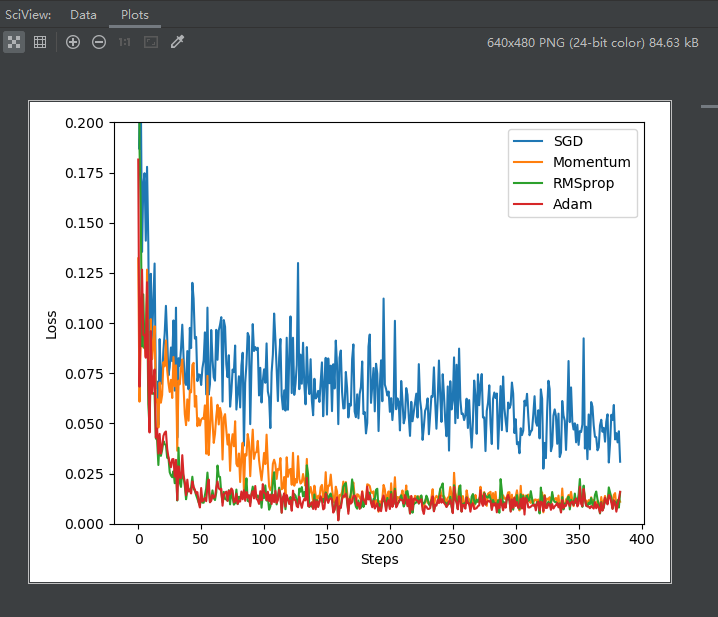### 四、PyTorch 数据处理工具箱

#### 4.1 数据处理工具箱概述

PyTorch 涉及数据处理（数据装载、数据预处理、数据增强等）

torch.utils.data 工具包，包括以下4个类：

1. Dataset : 是一个抽象类，其他数据集需要继承这个类，并且覆写其中的两个方法

     __getitem__  、   __len__


3. random_split : 把数据集随机拆分为给定长度的非重叠的新数据集。

4. *sampler : 多种采样函数

                  | ----->  Dataset   ------------->>   |-->datasets.(MNIST,)
|       __getitem__                   |                                 Pretrained = True
|        __len__                      |-->models.(AlexNet,ImageFolder,) ---------------->> torch.utils.model_zoo
|                                     |
|                                     |
|       (dataset,batch,)      torchvision(有四个功能模块) --> transforms.compose,
|                                        |
|------>  random_split                   |--> utils.make_grid,save_image
|
|------>  *sampler


Torchvision 是 PyTorch可视化处理工具，其是 PyTorch 的一个视觉处理工具包，独立于PyTorch，需要另外安装，使用 pip 或者 conda 安装即可：

pip install torchvision # 或者 conda install torchvision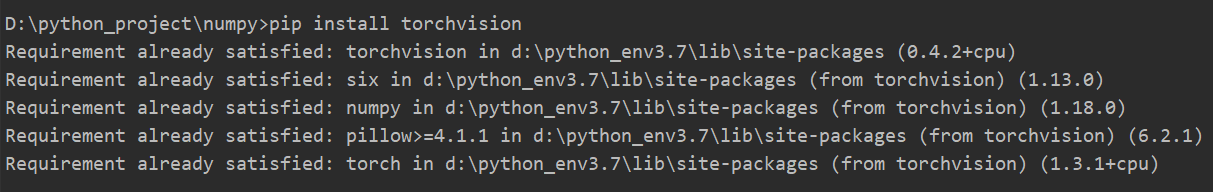torchvision 它包括4个类，各类的主要功能如下：

1. datasets : 提供常用的数据集加载，设计上都是继承自 torch.utils.data.Dataset，主要包括 MMIST、CIFAR10/100、ImageNetCOCO
2. models : 提供深度学习中各种经典的网络结构以及训练好的模型（如果选择 pretrained=True），包括 AlexNet、VGG系列、ResNet系列、Inception系列等。
3. transforms : 常用的数据预处理操作，主要包括对 Tensor 及 PIL Image 对象的操作。
4. utils : 含两个函数，一个是make_grid，它能将多张图片拼接在一个网络中；另一个是 save_img，它能将Tensor保存成图片

#### 4.2 utils.data 简介

torch.utils.data.Dataset 为 抽象类

__len__（提供数据大小 size）

1. 导入需要的模块

import torch
from torch.utils import data
import numpy as np

2. 定义获取数据集的类

class TestDataset(data.Dataset):   # 继承Dataset
def __init__(self):
self.Data=np.asarray([[1,2], [3,4], [4,5]])   # 一些由2维向量表示的数据集
self.Label=np.asarray([0,1,0,1,2])            # 这是数据集对应的标签

def __getitem__(self, index):
# 把 numpy 转换成 Tensor
txt=torch.from_numpy(self.Data[index])
label=torch.tensor(self.Label[index])
return txt, label

def __len__(self):
return len(self.Data)

3. 获取数据集中的数据

Test=TestDataset()
print(Test)   # 相当于调用__getitem__(2)
print(Test.__len__())


##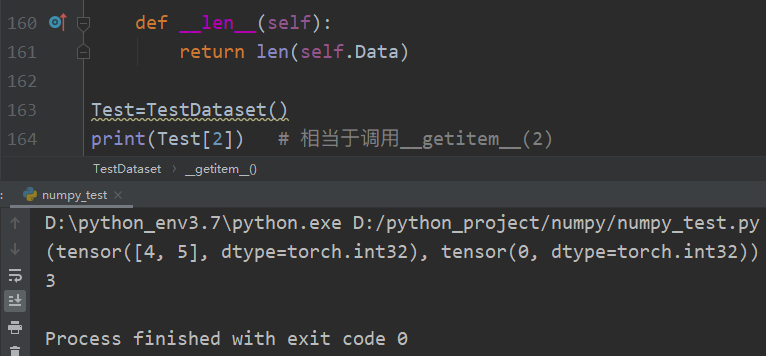test_loader = data.DataLoader(Test, batch_size=2, shuffle=False, num_worker=2)
print('i:',i)
Data,Label=traindata
print('data:', Data)
print('Label:', Label)


#### 4.3 torchvision 简介

##### –transforms

transforms 提供了对 PIL Image对象Tensor对象 的常用操作。

##### –ImageFolder

from torchvision import transforms,utils
from torchvision import datasets
import torch
import matplotlib.pyplot as plt

my_trans = transforms.Compose([
transforms.RandomResizedCrop(224),
transforms.RandomHorizontalFlip(),
transforms.ToTensor()
])
train_data = datasets.ImageFolder('./data/torchvision_data', transform = my_trans)

if i_batch == 0:
print(img)
fig = plt.figure()
grid = utils.make_grid(img)
plt.imshow(grid.numpy().transpose((1, 2, 0)))
plt.show()
utils.save_image(grid,'test01.png')
break


#### – tensorboardX简介

1. 导入tensorboardX，实例化SummaryWriter类，指明记录日志路径等信息。

from tensorboardX import SummaryWriter

# 实例化SummaryWriter，并指明日志存放路径。在当前目录没有logs目录将自动创建
writer = SummaryWriter(log_dir = 'logs')

# 调用实例

# 关闭writer
writer.close()

• 如果是Windows环境，log_dir注意路径解析，如：

writer = SummaryWriter(log_dir = r 'D:\myboard\test\logs')

• SummaryWriter的格式为：

SummaryWriter（log_dir=None, comment=' ', **kwargs）
# 其中comment在文件命名加上comment后缀

• 如果不写log_dir，系统将在当前目录创建一个runs的目录

1. 调用相应的API接口，接口一般格式为：

add__xxx(tag-name, object, iteration-number)

2. 启动 tensorboard 服务

cd 到 logs目录 所在的同级目录，在命令行输入如下命令， logdir等式右边可以是相对路径 或 绝对路径。
tensorboard --logdir=r'D:\myboard\test\logs' --port 6006

3. Web展示

在浏览器输入：
http://服务器IP 或者 名称：6006    # 如果是本机，服务器名称可以使用localhost


#### – 用tensorboardX可视化神经网络

1. 导入需要的模块

import torch
import torch.nn as nn
import torch.nn.functional as F
import torchvision
from tensorboardX import SummaryWriter

2. 构建神经网络

class Net(nn.Module):
def __init__(self):
super(Net, self).__init__()
self.conv1 = nn.Conv2d(1, 10, kernel_size=5)
self.conv2 = nn.Conv2d(10, 20, kernel_size=5)
self.conv2_drop = nn.Dropout2d()
self.fc1 = nn.Linear(320, 50)
self.fc2 = nn.Linear(50, 10)
self.bn = nn.BatchNorm2d(20)

def forward(self, x):
x = F.max_pool2d(self.conv1(x),2)
x = F.relu(x) + F.relu(-x)
x = F.relu(F.max_pool2d(self.conv2_drop(self.conv2(x)), 2))
x = self.bn(x)
x = x.view(-1, 320)
x = F.relu(self.fcl(x))
x = F.dropout(x, training=self.training)
x = self.fc2(x)
x = F.softmax(x, dim=1)
return x

3. 把模型保存为 graph

# 定义输入
input = torch.rand(32, 1, 28, 28)

# 实例化神经网络
model = Net()

# 将model 保存为 graph
with SummaryWriter(log_dir='---路径---', comment='Net') as w:


#### – 用tensorboardX可视化特征图

11-299万+

#### PyTorch 深度学习:60分钟快速入门12-111万+

#### 基于PyTorch的深度学习入门教程（五）——训练神经网络分类器

11-3014万+

#### 基于PyTorch的深度学习入门教程（一）——PyTorch安装和配置

11-017937

#### 基于pytorch的 保存和加载模型参数

07-01251

#### Python深度学习基于Pytorch

12-111万+

#### 基于PyTorch的深度学习入门教程（二）——简单知识

12-111万+

#### 基于PyTorch的深度学习入门教程（四）——构建神经网络©️2020 CSDN 皮肤主题: 书香水墨 设计师: CSDN官方博客点击重新获取扫码支付1.余额是钱包充值的虚拟货币，按照1:1的比例进行支付金额的抵扣。
2.余额无法直接购买下载，可以购买VIP、C币套餐、付费专栏及课程。余额充值